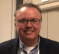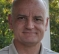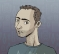## Computing the delay from a room response FIR filterStarted by 4 years ago9 replieslatest reply 4 years ago323 views

Hello,

I'm trying to figure out the best way to compute the delay from a room response filter. This room response filter was measured by playing white noise from speakers and recording at a microphone where the transfer function is of interest. The context is that the input filtered with this room response will then be the reference signal for an echo canceller (adaptive NLMS filter) and needs to be delayed appropriately to match the echo on the microphone.

Now, a linear phase FIR filter's delay is N/2 where N is the number of taps in the FIR filter. However an impulse response has a peak around the delay between the speaker and mic, plus it has energy at other taps due to reflections/noise/etc. In a clean system, an impulse response with value unity at the delay tap and zero elsewhere will simply delay the input by delay_samples.

However, the room response/impulse response in practice is neither a linear phase filter, nor a pure delay system as above. So it won't have a constant group delay. As a result, different frequencies get delayed by different amounts. Just to illustrate this in a crude way, I just modified the filter with gradually decaying values - it's not a real impulse response, but just an example to illustrate the question.

I need to find a way to put a number to the delay from such a room response filter to use further downstream. Crosscorrelation between the input and output shows a delay of 6 samples. This kind of makes sense given that the peak is still around 5, and the other samples act on stretching the delay out further. As a result, the larger the amplitude of the impulse response at longer taps, the larger the overall delay would be. In essence it is a weighted average with the predominant weight corresponding to the actual delay between the speaker and mic used to measure this impulse response.

However, is that good way to characterize the delay introduced by this filtering, given the need to estimate the delay for subsequent adaptive filtering for echo cancellation?

Thank you!

[ - ]I will use frequency sweep signal instead of white noise, of bandwidth equal to my system bandwidth. compare DFT of input and output as ratio, get phase of this ratio, now representing my system response and convert phase to group delay. It will show how it varies with frequency.

[ - ]Thaks @kaz.

Could you tell me why the sine sweep is better than white noise?

Also, I tried your method (still with white noise) for estimating the group delay by taking the negative derivative of phase, but it doesn't seem to yield the same answer as the grpdelay function in MATLAB. Can you suggest a reliable way to compute the grpdelay from phase in MATLAB?

[ - ]You can use white noise but I prefer a clean frequency sweep for measurement. Here is how I will do example model system (iir) from frequency sweep input and compare it to matlab freqz function:

Run this code and compare amp1,amp2 & gd1, gd2

---------------------------------------------------

n = 2^12;   %resolution

x = cos(2*pi*(0:n-1).*linspace(0,.25,n));   %chirp test input

num = .3;       %iir model

den = [1, -.7];

%method1 , iir frequency response using freqz

[H,f] = freqz(num,den,linspace(0,1,n),1);

amp1= abs(H);

gd1 = [-diff(unwrap(angle(H)))./diff(2*pi*f), 0]; %groupdelay

%method2: fft ratio

y = filter(num,den,x); %apply filter to test input

fftx = fft(x);

ffty = fft(y);

fft_ratio = ffty./fftx; %get fft ratio of output to input

%amplitude and group delay from fft ratio

amp2 = abs(fft_ratio);

Amp2 = amp2/max(amp2); %normalise fft scaling

gd2 = [-diff(unwrap(angle(fft_ratio)))./diff(2*pi*f), 0];

-----------------------------------------------------------

[ - ]Awesome! Thank you!
gd1 and gd2 are the same and they also match with MATLAB's built-in grpdelay function which can be used directly on [num,den] coefficients.

[ - ]Late to the party...

I prefer multisines.  A good classic paper on excitation sequences is this:

J. Schoukens, R. Pintelon, E. van der Ouderna, J. Renneboog, “Survey of Excitation Signals for FFT Based Signal Analyzers”, IEEE Trans. Instrum. Meas., vol. 37, September 1988, pp. 342–352

The issue - mostly - is balancing spectral density with crest factor.

Spectral density - Frequency content vs time.

Crest factor - Peak to rms ratio.

High crest factor excitations - among other things - excite nonlinearities.

An impulse gives you amazing spectral density with high crest factor.  A random sequence has lower crest factor, but you might die of old age before your particular frequency content needs are satisfied.  ...and so on.

[ - ]Hi planb_buzz,

If your measured delay is only 6 samples, and you are looking at a room impulse response of, probably, hundreds of samples, then I don't understand why you should care about the 6 samples.
The echo canceller will learn that those taps should be 0 anyway.

In my view, there are only three significant sources of delay for the problem that you outline, and the first two seem to be outside the issue you are facing.
1) The buffering of the samples going to the speaker
2) The buffering of the samples coming from the mic
3) The physical delay between the speaker and the mic

The buffer delays should be fixed and remain predictable.
The physical delay can also be calculated from the distance between the mic and speaker and using the speed of sound (~343 m/s) to figure out how many samples that should be.
It is fairly straight forward to calculate all of this if you really feel that you need to compensate.

As a slight aside, most acoustic echo cancellation is done in the frequency domain these days for some important reasons.
1) The required processing can be reduced by a factor of approximately the number of sub-bands you choose to use.
2) The processing resources can be distributed to deal with impulse response length vs. frequency.  The low frequencies, which have much longer impulse responses because reverb, can use much longer filters, and high frequencies can have much shorter filters.
3) Convergence can be much faster and more predictable, because each sub-band sees a flatter spectrum, and because the step size in each band can be "normalized" (NLMS) based on the energy within that band vs. over the entire spectrum.

Another observation, based on you question, is that almost nothing in the real world is linear phase and room IRs are a prime example of this.  In fact, most real world things are much closer to minimum phase in their response.

Regards,

David

[ - ]Hello David,

The 6 samples delay was just an example to illustrate the question. The real latencies I am dealing with are on the order of 500 samples at 48kHz (i.e. 10ms). While the echo cancellation will accurately come up with zero weights for those taps, it is still a wastage of a lot of filter taps. So, the goal is to apply delay to the reference signal to maximize the use of the adaptive filter taps.

Also, the room response filtering as well as the echo cancellation is indeed in the subband domain, but I am trying to break down the problem to estimating delays required to be applied to the reference signal. I could always plot the subband adaptive filter coefficients and see if they catch the echo correctly and work back to adjust the delay. But I was interested in deterministically computing this. When I started thinking about this, it led me to think about what the impact of a non-linear phase filter was on delay when a subsequent module cares about the delay. It seems like the only deterministic aspect is that there will be a non-flat group delay and the echo delay for the adaptive filter might just have to accommodate the delay of the largest frequency as shown in the grpdelay calculations.

Thanks!

[ - ]Aha! Nice work.
My bet is that the 500 or so samples of delay are dominated by the buffering.  If we guess 480 samples, that is 10 msec at 48k sampling.

My first suggestion then is to figure out those buffers, and the consistency of that delay.

Try just running the signal directly from the speaker out into the mic in with some attenuation.  The delay should be close to a some multiple of 5 msec for most audio systems, on either phones or PCs, because the buffering is usually set up in a way related to the encoding or decoding of speech or music. They are almost always processed in multiples of 5 msec.

Having figured that out, you can just delay your reference signal by the right integer number of buffers.

Hopefully the delay is consistent.  Good luck.

David

[ - ]